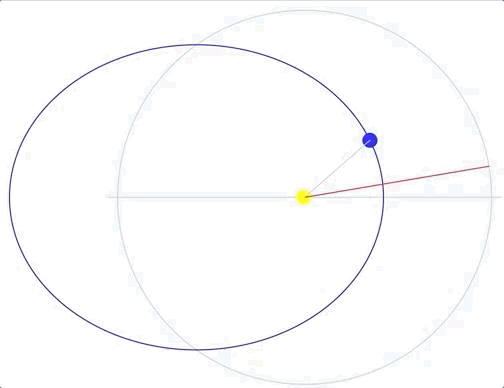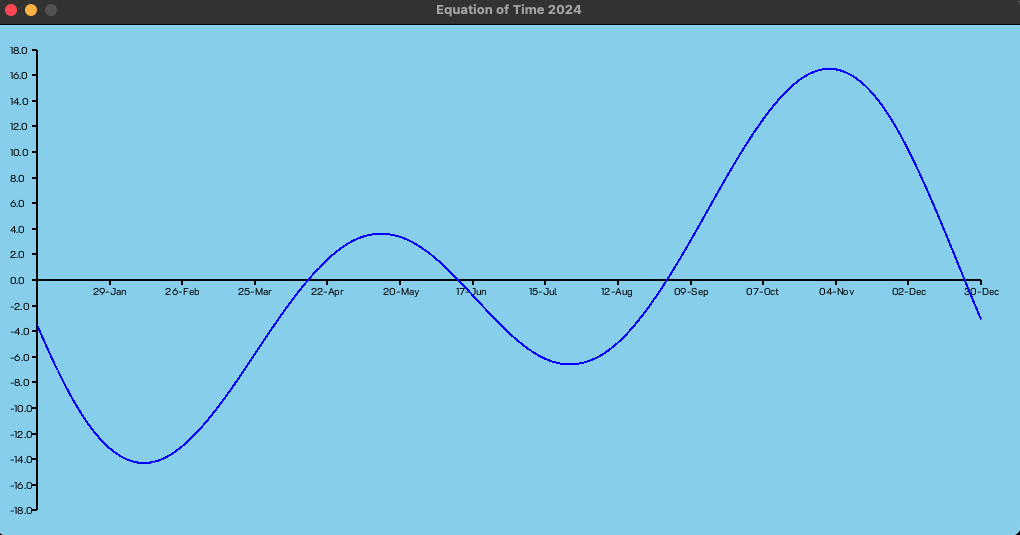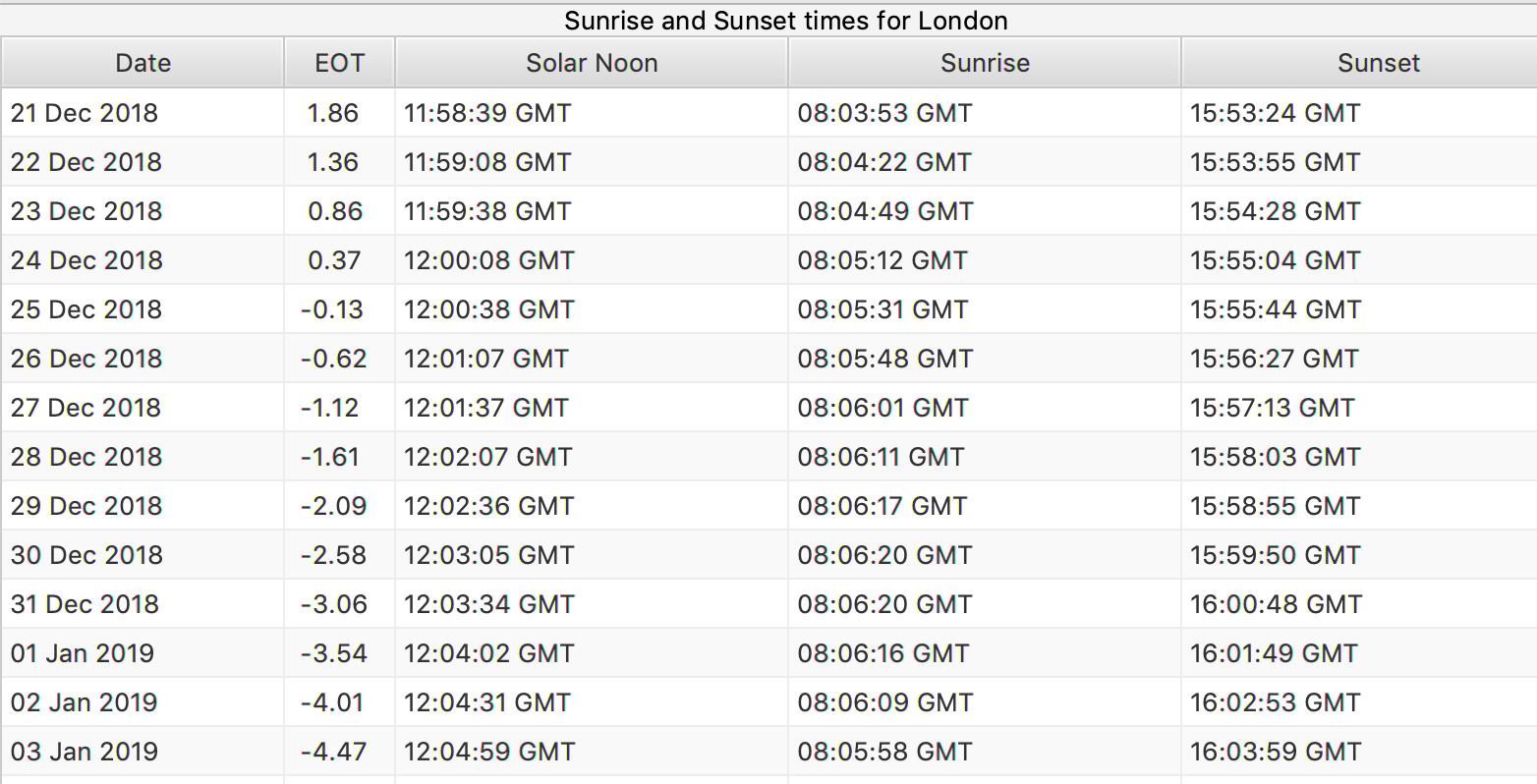# Dr Phill's Science Made Simple

## Equation of Time

The question is, why are sunrise and sunset not symmetrical about noon most days? This effect is most apparent around the December Solstice when, in the Northern Hemisphere, sunrise is about the same time for several weeks, but sunset gets later every day. Time was traditionally measured using the position of the Sun or the positions of stars. Solar noon is the time when the Sun is at its highest position in the sky. When clocks were invented it was found that solar noon and clock noon rarely agree. The explanation of why solar noon and clock noon differ is called the Equation of Time. In fact solar noon and clock noon only agree on four days per year.

Modern clocks assume every day is exactly 24 hour long. If the Earth's orbit was perfectly circular and the Earth's rotation axis was perpendicular to its orbital plane, solar noon would agree with clock noon every day. The Sun however, doesn't culminate at exactly noon clock time every day due to the fact that the solar day varies in length from day to day due to orbital effects. The difference between clock time and solar time is described by the equation of time. The Equation of Time is usually displayed as a graph. Some sundials have the Equation of Time as a correction table. The Equation of Time shows the cumulative difference between clock time and solar time. It has two major components. One is due to the Earth's orbit being elliptical. The second is due to the Earth's axial tilt. There are also some much smaller components due to the gravitational pull of other planets and due to relativistic effects.

### Longitude

The actual time solar noon occurs is also dependent upon the longitude and timezone of the observer. Solar noon can only occur at 1200 if the observer is at a longitude which is a multiple of 15°. It also requires that the time zone of the observer is aligned with the longitude. For the purpose of this discussion it will be assumed that the observer is on the Greenwich Meridian 0° longitude and the clock is set to Greenwich Mean Time (GMT).

### Orbital Eccentricity

When the Earth is at perihelion, its closest point to the Sun, it is travelling at its fastest. When the Earth is at aphelion, its furthest point from the Sun, it is travelling at is slowest. The time difference between two successive perihelia is called the anomalistic period. If we ignore the other components, this means that solar noon and clock noon only coincide at the apses. The amimation shows this effect. The red line shows clock time and the planet shows solar time.Johannes Kepler published his three laws which descibe planetary orbits. His first law states that orbits are ellipses with the Sun at one of the foci. He defined the mean anomaly M to be the angle from perihelion to the position the planet would be if its orbit was circular. It sweeps out the same angle for the same time increment. This corresponds to clock time. Kepler's second laws states that a line joining a planet and the Sun sweeps out equal areas during equal intervals of time. The true anomaly ν is the angle from perihelion to the actual position of the planet. Kepler showed how to calculate the true anomaly from the mean anomaly.

The equation of centre is defined to be the difference in angle between the true anomaly and the mean anomaly. The eccentricity component of the equation of time is minus the equation of centre calculated at clock noon converted into a day angle in minutes.

$$\Delta T = \frac{(M - \nu) \cdot 24 \cdot 60}{2\pi}$$

A negative value means that solar noon occurs before clock noon and a positive value means that solar noon is after clock noon.

The Kepler calculation is a good approximation to the value of the equation of time component. It is used in most descriptions of the equation of time. The calculations assume that the position, and hence time, of perihelion is fixed and the obit is perfectly elliptical. Neither of these are true in reality. The time of perihelion is precessing. It is getting progressively later by about a day every 58 years. The shape of the Earth's orbit is contantly changing due to the gravitational effects of the other planets.

Using NASA JPL Ephemerides data it is possible to calculate the position of the Sun, Moon and planets, including Pluto, to a high degree of accuracy for any time between the years 1550 and 2550. It is possible to calculate the time of perihelion and aphelion for any given year using iterative techniques to find the nearest and farthest distance from the Sun. Perihelion currently occurs around the 3rd of January. Interestingly the actual time of perihelion varies from year to year and can occur on the 2nd, 3rd, 4th or 5th of January.

The eccentricity component of the equation of time can be calculated directly from Earth position data. The times of the apses can be calculated accurately. It is also known that the mean anomaly and true anomaly are the same at the apses. Given that a perihelion accurs at time $$t_p$$ and longitude $$\lambda_p$$ and the next aphelion occurs at time $$t_a$$ and longitude $$\lambda_a$$ the mean anomaly and true anomaly can be calculated during that orbit section. The mean anomaly at time t is:

$$M = \frac{t - t_p}{t_a - t_p}\pi$$

The true anomaly which is at longitude λ at time t is:

$$\nu = \frac{\lambda - \lambda_p}{\lambda_a - \lambda_p}\pi$$

The mean anomaly and true anomaly can be calculated for an orbit section between an aphelion and the following perihelion by reversing the perihelion and aphelion terms and adding π.

The eccentricity component is a sine like curve with a period of an anomalistic year. It has zeros at the apses and an amplitude of 7.69 minutes.

### Obliquity

The obliquity component of the Equation of Time is the result of the Earth's axis of rotation being inclined at an angle of about 23°. When the Sun's poition is projected onto the equatorial plane its longitude shifts. This means that the Sun's position at high noon is shifted and appears earlier or later.

At a time t, which is a clock midday, the Earth has a longitude λ. The shift in longitude α caused the the Earth's obliquity angle φ is given by $$\tan\alpha = \cos\phi\tan\lambda$$. Then the obliquity equation of time component is the difference of the two angles converted into a day angle in minutes.

$$\Delta t = \frac{(\lambda - \alpha) \cdot 24 \cdot 60}{2\pi}$$

The eccentricity component is a sine like curve with a period of the time between equinoxes. It has zeros at the equinoxes and solstices and an amplitude of 9.86 minutes.

### Equation of Time

The Equation of Time is calculated by adding the two components as shown in the diagram.